前言

👧美术小姐姐：皮皮皮皮，你能不能做奶茶？

😱我：？？？

👧美术小姐姐：就是那种，奶茶的轮廓加上动态水波纹~

🙄我：吓死我还以为让我做喝的奶茶...

👧美术小姐姐：炒鸡多图片都需要这种效果，用动画的话工作量太大了！

🤓我：波浪效果是吧，小意思，一个月的奶茶就够了，或者扫码提需求~

👧美术小姐姐：皮？🔨🔨🔨

🤪我：卒~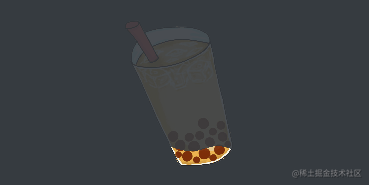正文

🧐整体思路

🔢正弦曲线（Sinusoid）

$y = \sin(x)$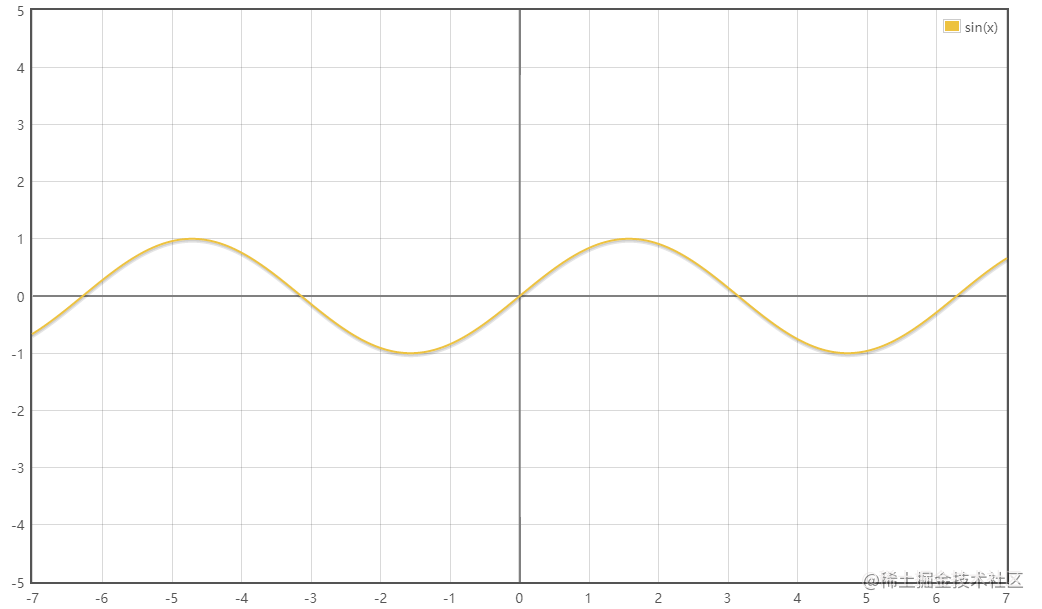$y = A \cdot \sin(\omega x ± \phi) + k$

• A振幅（Amplitude），曲线最高点与最低点的差值，表现为曲线的整体高度
• ω角速度（Angular Velocity），控制曲线的周期，表现为曲线的紧密程度
• φ初相（Initial Phase），即当 x = 0 时的相位，表现为曲线在坐标系上的水平位置
• k偏距（Offset），表现为曲线在坐标系上的垂直位置

☕稍加思索

改变曲线的高度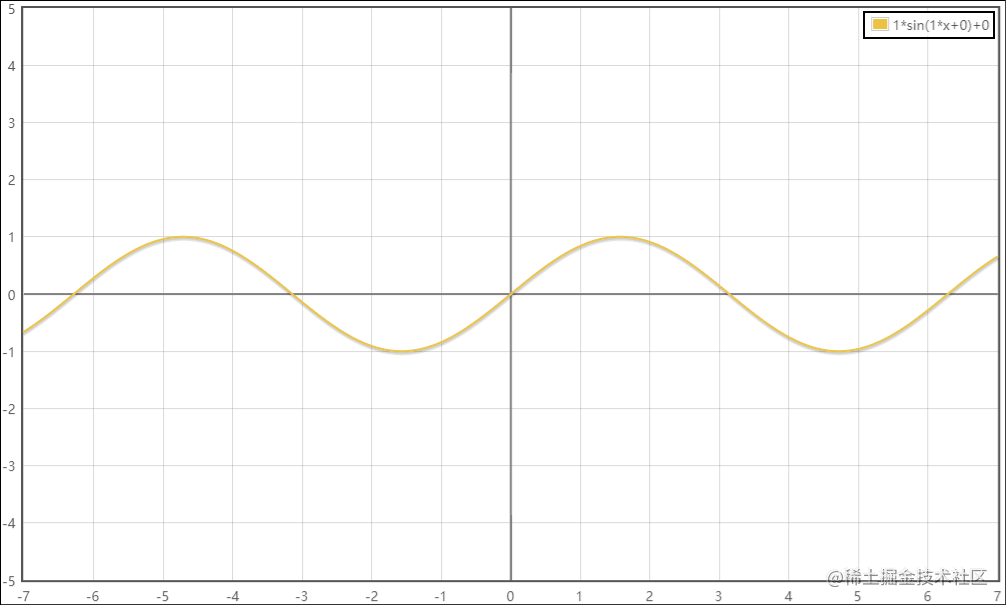改变曲线的周期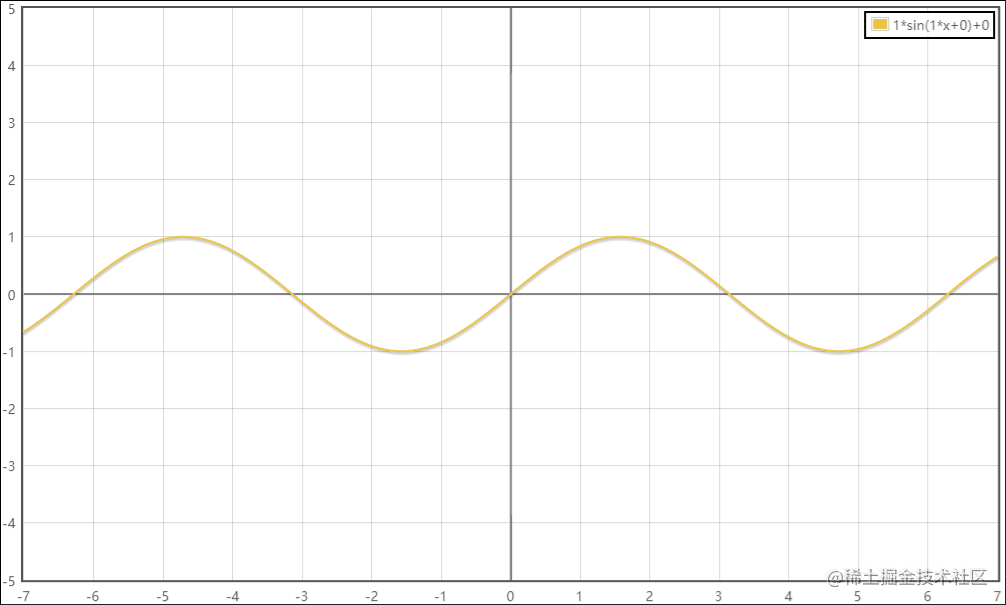改变曲线的水平位置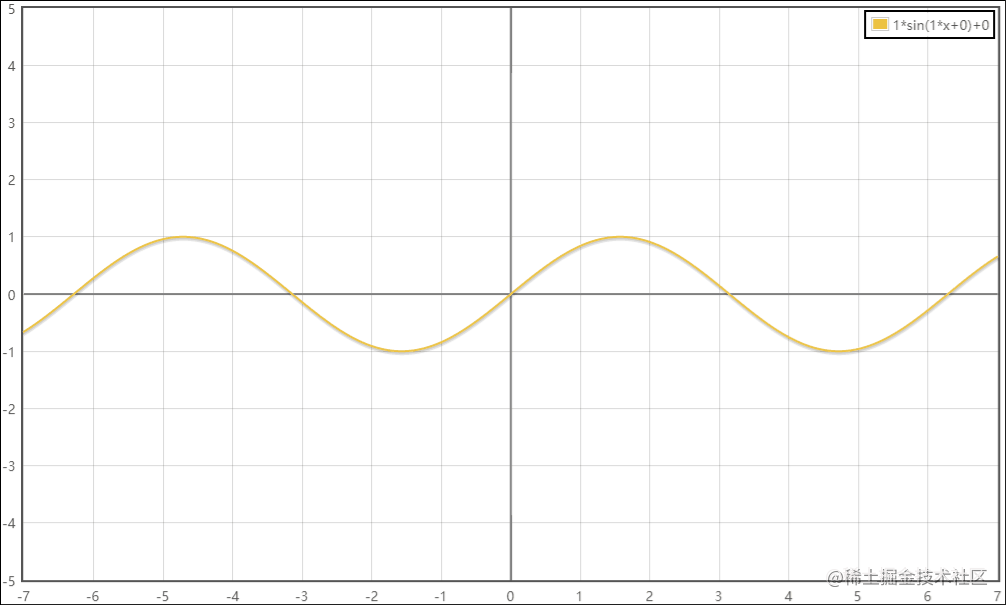改变曲线的垂直位置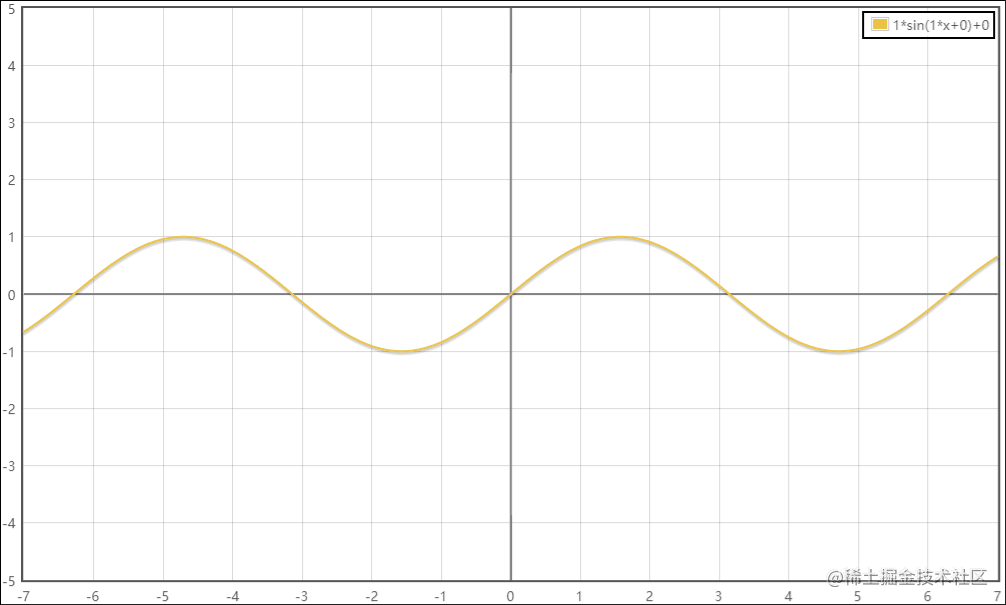🤠动手实现

🌊如何让曲线动起来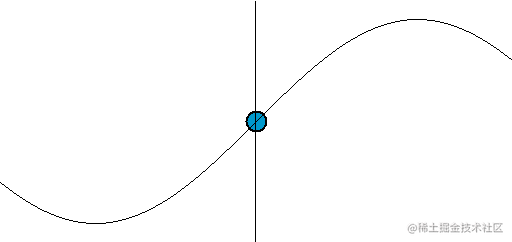得到新的公式

$y = A \cdot \sin(\omega x ± \phi t) + k$

void mainImage( out vec4 fragColor, in vec2 fragCoord )
{
// 将像素坐标归一化（区间 [0.0, 1.0]）
vec2 uv = fragCoord / iResolution.xy;

// 振幅（控制波浪顶端和底端的高度）
float amplitude = 0.05;

// 角速度（控制波浪的周期）
float angularVelocity = 10.0;

// 频率（控制波浪移动的速度）
float frequency = 10.0;

// 偏距（设为 0.5 使得波浪垂直居中于屏幕）
float offset = 0.5;

// 初相位（正值表现为向左移动，负值则表现为向右移动）
float initialPhase = frequency * iTime;

// 代入正弦曲线公式计算 y 值
// y = Asin(ωx ± φt) + k
float y = amplitude * sin((angularVelocity * uv.x) + initialPhase) + offset;

// 区分 y 值上下部分，设置不同颜色
vec4 color = uv.y > y ? vec4(0.0, 0.0, 0.0, 1.0) : vec4(0.0, 0.7, 0.9, 1.0);

// 输出到屏幕
fragColor = color;
}🥥On Cocos Creator

Effect 文件：gitee.com/ifaswind/ea…

CCProgram fs %{
precision highp float;

// 引入 Cocos Creator 内置的全部变量
#include <cc-global>

// 顶点颜色（来自顶点着色器）
in vec4 v_color;
// UV 坐标（来自顶点着色器）
in vec2 v_uv0;

// 纹理
uniform sampler2D texture;

// 自定义属性
uniform Properties {
float amplitude;		// 振幅
float angularVelocity;	// 角速度
float frequency;		// 频率
float offset;			// 偏距
};

void main () {
// 保存顶点颜色
vec4 color = v_color;

// 叠加纹理颜色
color *= texture(texture, v_uv0);

// 直接丢弃原本就透明的像素

// 初相位（正值表现为向左移动，负值则表现为向右移动）
// cc_time 是 Cocos Creator 提供的运行时间全局变量（类型：vec4）
float initiaPhase = frequency * cc_time.x;

// 代入正弦曲线公式计算 y 值
// y = Asin(ωx ± φt) + k
float y = amplitude * sin(angularVelocity * v_uv0.x + initiaPhase) + offset;

// 丢弃 y 值以上的像素（左上角为原点 [0.0, 0.0]）

// 输出颜色
gl_FragColor = color;
}
}%cc.tween(this.sineWave)
.to(3, { height: 1 })
.to(0.5, { amplitude: 0 })
.start();SineWave 组件：gitee.com/ifaswind/ea…

传送门

Eazax-CCC 游戏开发脚手架

Eazax-CCC 示例在线预览

更多分享

《为什么选择使用 TypeScript ？》

《一文看懂 YAML》

《Cocos Creator 性能优化：DrawCall》

《互联网运营术语扫盲》

《在 Cocos Creator 里画个炫酷的雷达图》

公众号

😺菜鸟小栈

💻我是陈皮皮，这是我的个人公众号，专注但不仅限于游戏开发、前端和后端技术记录与分享。

💖每一篇原创都非常用心，你的关注就是我原创的动力！

Input and output.